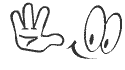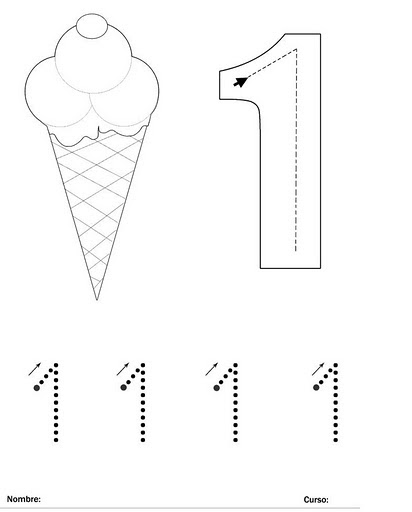• NCERT नवा अभ्यासक्रमनु BEST मटेरियल ALL IN ONE
• #Like Facebook Page

## 17 June 2020

### 1 TO 100 NUMBER Tracing Learning and Writing one to 100

A number is a mathematical object used to count, measure, and label. The original examples are the natural numbers 1, 2, 3, 4, and so forth. For being manipulated, individual numbers need to be represented by symbols, called numerals; for example, "5" is a numeral that represents the number five. As only a small number of symbols can be memorized, basic numerals are commonly organized in a numeral system, which is an organized way to represent any number. The most common numeral system is the Hindu–Arabic numeral system, which allows representing any number by a combination of ten basic numerals called digits.
Kid's Can learn by tracing and counting numbers and things given in each page of this tracing book.